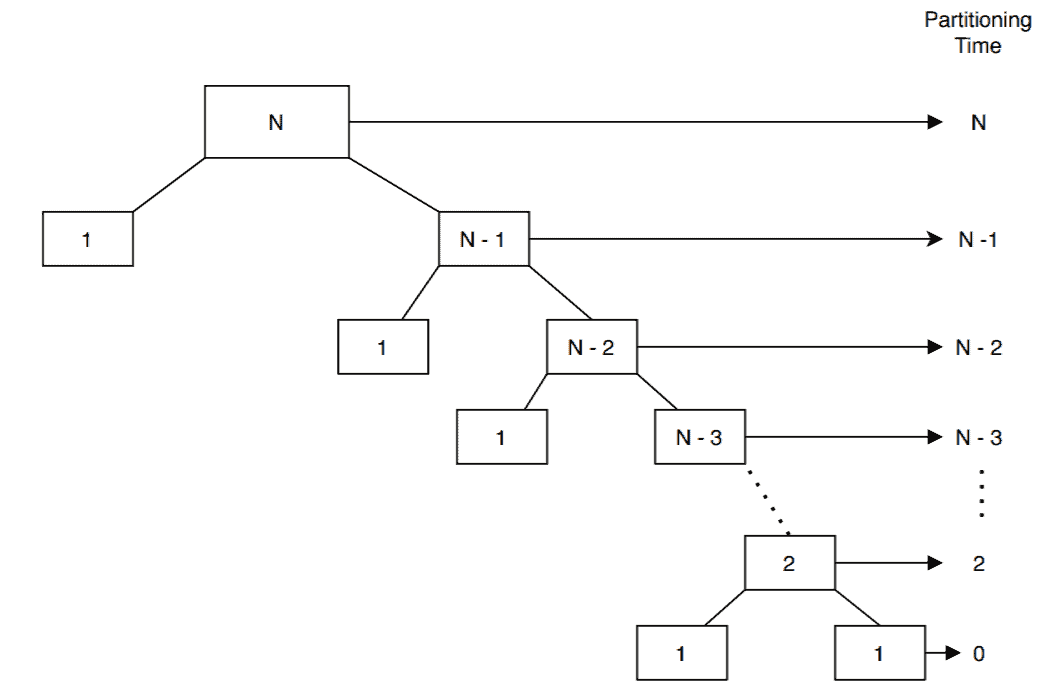## 1. Overview

An efficient sorting algorithm plays an important role in reducing the complexity of a problem. Sorting algorithms are used in various problems in computer science to rearrange the elements in an input array or list in ascending or descending order.

Quicksort is a highly efficient sorting that is based on the Divide-and-Conquer method.

In this tutorial, we’ll discuss the worst-case scenario for the Quicksort algorithm in detail.

## 2. When Does the Worst Case of Quicksort Occur?

The efficiency of the Quicksort algorithm very much depends on the selection of the pivot element. Let’s assume the input of the Quicksort is a sorted array and we choose the leftmost element as a pivot element. In this case, we’ll have two extremely unbalanced arrays. One array will have one element and the other one will haveelements.

Similarly, when the given input array is sorted reversely and we choose the rightmost element as the pivot element, the worst case occurs. Again, in this case, the pivot elements will split the input array into two unbalanced arrays.

Except for the above two cases, there is a special case when all the elements in the given input array are the same. In such a scenario, the pivot element can’t divide the input array into two and the time complexity of Quicksort increases significantly.

## 3. Worst Case Time Complexity Analysis

Let’s assume that we choose a pivot element in such a way that it gives the most unbalanced partitions possible:All the numbers in the box denote the size of the arrays or the subarrays.

Let’s consider an input array of size. The first partition call takestimes to perform the partition step on the input array.

Each partition step is invoked recursively from the previous one. Given that, we can take the complexity of each partition call and sum them up to get our total complexity of the Quicksort algorithm.

Therefore, the time complexity of the Quicksort algorithm in worst case isAlternatively, we can create a recurrence relation for computing it.

In the worst case, after the first partition, one array will haveelement and the other one will haveelements. Let’s saydenotes the time complexity to sortelements in the worst case:Again for the base case whenand, we don’t need to sort anything. Hence, the sorting time isandNow, we’re ready to solve the recurrence relation we derived earlier:,,,,,,,As a result,## 4. Avoiding the Worst Case

We can avoid the worst-case in Quicksort by choosing an appropriate pivot element. In this section, we’ll discuss different ways to choose a pivot element.

The first approach for the selection of a pivot element would be to pick it from the middle of the array. In this way, we can divide the input array into two subarrays of an almost equal number of elements in it.

In some cases selection of random pivot elements is a good choice. This variant of Quicksort is known as the randomized Quicksort algorithm.

Another approach to select a pivot element is to take the median of three pivot candidates. In this case, we’ll first select the leftmost, middle, and rightmost element from the input array. Then we’ll arrange them to the left partition, pivot element, and right partition.

Quicksort is considered one of the best sorting algorithms in terms of efficiency. The average case time complexity of Quicksort iswhich is the same as Merge Sort. Even with a large input array, it performs very well. It provides high performance and is comparatively easy to code. It doesn’t require any additional memory.

The main disadvantage of quicksort is that a bad choice of pivot element can decrease the time complexity of the algorithm down to. Also, it’s not a stable sorting algorithm.

## 6. Implementation

To see Quicksort in practice please refer to our Quicksort in Java article.

## 7. Conclusion

In this tutorial, we discussed the different worst-case scenarios of Quicksort and presented the time complexity analysis for it.